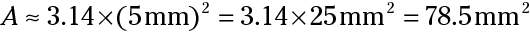##### Basic Math & Pre-Algebra For Dummies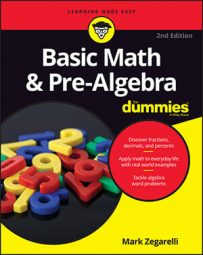The center of a circle is a point that's the same distance from any point on the circle itself. This distance is called the radius of the circle, or r for short. And, any line segment from one point on the circle through the center to another point on the circle is called a diameter, or d for short.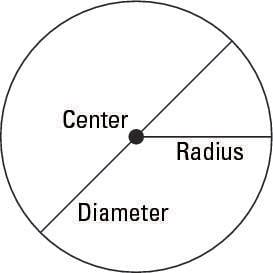## The diameter

As you can see, the diameter of any circle is made up of one radius plus another radius — that is, two radii (pronounced ray-dee-eye). This concept gives you the following handy formula: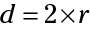For example, given a circle with a radius of 5 millimeters, you can figure out the diameter as follows: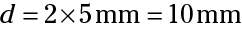## The circumference

Because the circle is an extra-special shape, its perimeter (the length of its "sides") has an extra-special name: the circumference (C for short). Early mathematicians went to a lot of trouble to figure out how to measure the circumference of a circle. Here's the formula they hit upon: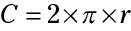Note: Because 2 x r is the same as the diameter, you also can write the formula as C = π x d.

The symbol π is called pi (pronounced "pie"). It's just a number whose approximate value is as follows (the decimal part of pi goes on forever, so you can't get an exact value for pi):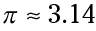So given a circle with a radius of 5 mm, you can figure out the approximate circumference: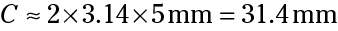## The area of a circle

The formula for the area (A) of a circle also uses π: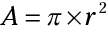Here's how to use this formula to find the approximate area of a circle with a radius of 5 mm: